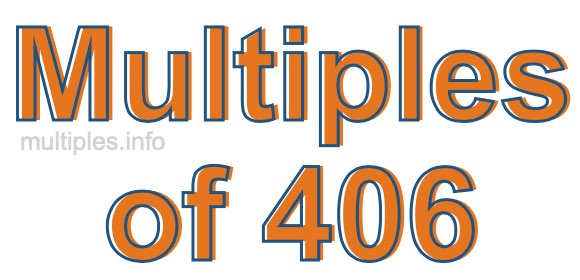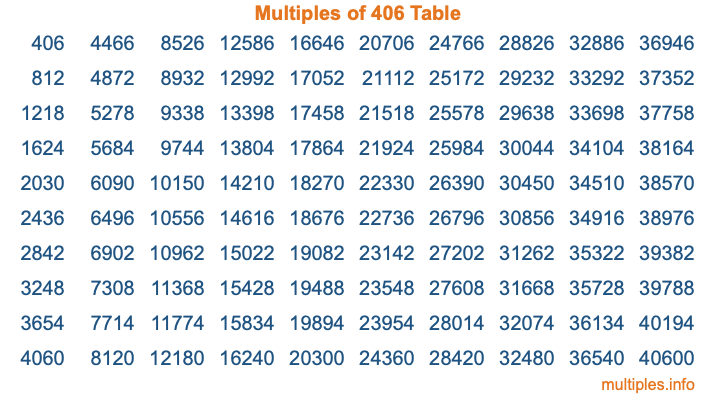Multiples of 406Welcome to the Multiples of 406 page. Here we will first teach you everything you will ever need to know about the multiples of 406, and then give you a study guide summary of everything we taught you to make sure you remember it all. Use this page to look up facts and learn information about the multiples of 406. This page will make you a multiples of four hundred six expert!

Definition of Multiples of 406
Multiples of 406 are all the numbers that when divided by 406 equal an integer. Each of the multiples of 406 are called a multiple. A multiple of 406 is created by multiplying 406 by an integer.

Therefore, to create a list of multiples of 406, you start with 1 multiplied by 406, then 2 multiplied by 406, then 3 multiplied by 406, and so on for as long as you want. Thus, the list of the first five multiples of 406 is 406, 812, 1218, 1624, and 2030. To see a larger list of multiples of 406, see the printable image of Multiples of 406 further down on this page. We also have a category where you can choose any nth multiple of 406.

Multiples of 406 Checker
The Multiples of 406 Checker below checks to see if any number of your choice is a multiple of 406. In other words, it checks to see if there is any number (integer) that when multiplied by 406 will equal your number. To do that, we divide your number by 406. If the the quotient is an integer, then your number is a multiple of 406.

Is  a multiple of 406?

Least Common Multiple of 406 and ...
A Least Common Multiple (LCM) is the lowest multiple that two or more numbers have in common. This is also called the smallest common multiple or lowest common multiple and is useful to know when you are adding our subtracting fractions. Enter one or more numbers below (406 is already entered) to find the LCM.

Check out our LCM Calculator if you need more details about the Least Common Multiple or if you need the LCM for different numbers for adding and subtraction fractions.

nth Multiple of 406
As we stated above, 406 is the first multiple of 406, 812 is the second multiple of 406, 1218 is the third multiple of 406, and so on. Enter a number below to find the nth multiple of 406.

th multiple of 406

Multiples of 406 vs Factors of 406
406 is a multiple of 406 and a factor of 406, but that is where the similarities end. All postive multiples of 406 are 406 or greater than 406. All positive factors of 406 are 406 or less than 406.

Below is the beginning list of multiples of 406 and the factors of 406 so you can compare:

Multiples of 406: 406, 812, 1218, 1624, 2030, etc.

Factors of 406: 1, 2, 7, 14, 29, 58, 203, 406

As you can see, the multiples of 406 are all the numbers that you can divide by 406 to get a whole number. The factors of 406, on the other hand, are all the whole numbers that you can multiply by another whole number to get 406.

It's also interesting to note that if a number (x) is a factor of 406, then 406 will also be a multiple of that number (x).

Multiples of 406 vs Divisors of 406
The divisors of 406 are all the integers that 406 can be divided by evenly. Below is a list of the divisors of 406.

Divisors of 406: 1, 2, 7, 14, 29, 58, 203, 406

The interesting thing to note here is that if you take any multiple of 406 and divide it by a divisor of 406, you will see that the quotient is an integer.

Multiples of 406 Table
Below is an image of the first 100 multiples of 406 in a table. The table is in chronological order, column by column. The first column has the first ten multiples of 406, the second column has the next ten multiples of 406, and so on.The Multiples of 406 Table is also referred to as the 406 Times Table or Times Table of 406. You are welcome to print out our table for your studies.

Negative Multiples of 406
Although not often discussed or needed in math, it is worth mentioning that you can make a list of negative multiples of 406 by multiplying 406 by -1, then by -2, then by -3, and so on, to get the following list of negative multiples of 406:

-406, -812, -1218, -1624, -2030, etc.

Multiples of 406 Summary
Below is a summary of important Multiples of 406 facts that we have discussed on this page. To retain the knowledge on this page, we recommend that you read through the summary and explain to yourself or a study partner why they hold true.

There are an infinite number of multiples of 406.

A multiple of 406 divided by 406 will equal a whole number.

406 divided by a factor of 406 equals a divisor of 406.

The nth multiple of 406 is n times 406.

The largest factor of 406 is equal to the first positive multiple of 406.

406 is a multiple of every factor of 406.

406 is a multiple of 406.

A multiple of 406 divided by a divisor of 406 equals an integer.

406 divided by a divisor of 406 equals a factor of 406.

Any integer times 406 will equal a multiple of 406.

Multiples of a Number
Here you can get the multiples of another number, all with the same attention to detail as we did for multiples of 406 on this page.

Multiples of
Multiples of 407
Did you find our page about multiples of four hundred six educational? Do you want more knowledge? Check out the multiples of the next number on our list!